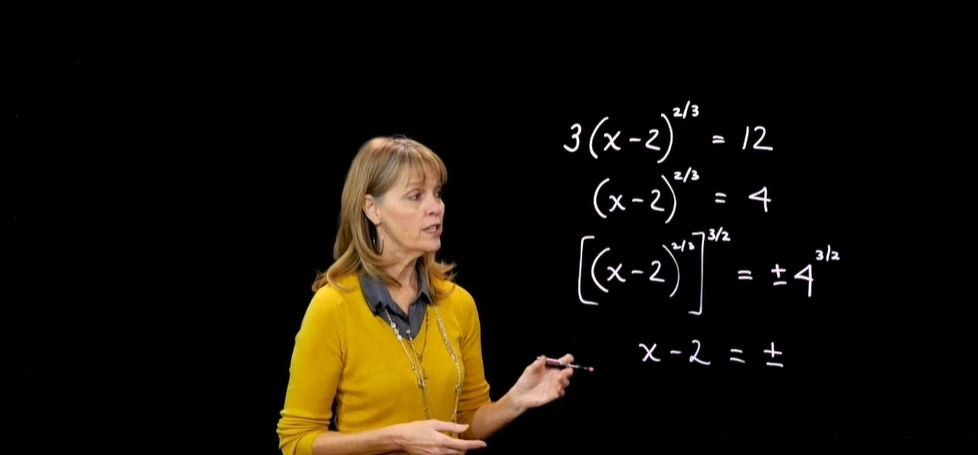Start typing, then use the up and down arrows to select an option from the list.
1:24 minutes
Problem 179
Textbook Question

# Exercises 177–179 will help you prepare for the material covered in the next section. If - 8 is substituted for x in the equation 5x^(2/3) + 11x^(1/3) + 2 = 0, is the resulting statement true or false?Verified Solution
This video solution was recommended by our tutors as helpful for the problem above.
26views